UPSC  >  Geometry Questions with Answer- 2

# Geometry Questions with Answer- 2 - CSAT Preparation - UPSC

Question 11: What is the ratio of longest diagonal to the shortest diagonal in a regular octagon?

A. √3 : 1

B. 2 : 1

C. 2 : √3

D. √2 : 1

Explanation.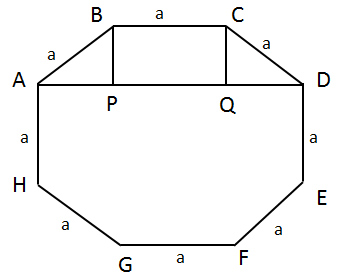Consider regular octagon ABCDEFGH
Its longest diagonal would be AE or BF or CG or DH.
Let us try to find out AE.
PQ = a
AP = QD
a2 = BP2 + AP2 => a2 = 2 AP2 {since BP=AP}
a = √2AP => AP = a/(√2)
AD =AP + PQ + QD = a/(√2) + a + a/(√2)
⇒ a + a√2
AE2 = (a + a√2) 2 + a2
AE2 = (a2 + 2 * a * √2 + 2a2) + a2
AE2 = a2 (1 + 2√2 + 2) + a2
⇒ a2 (4 + 2√2)
Shortest diagonal = AC or CE
AC2 = AB2 + BC2 – 2AB × BC cos135 degree
(Alternatively, we can deduce this using AC2 = AQ2 + QC2. We use cosine rule just to get some practice on a different method.)
= a2 + a2 – 2a2 * ((−1)/√2)
= 2a2 + √2a2
= a2 (2 + √2)
AE2 = a2 (4 + 2√2)
AE2/AC2 = a2 (4 + 2√2) / a2 (2 + 2√2) = 2
AE / AC = √2
Remember, for a regular octagon.
Each internal angle = 135 degrees.
Each external angle = 45 degrees.
So, we get a bunch of squares and isosceles right–angled △s if we draw diagonals.
A regular hexagon breaks into equilateral triangles. A regular octagon breaks into isosceles right angled triangles.

The question is "What is the ratio of longest diagonal to the shortest diagonal in a regular octagon?"

##### Hence, the answer is √2 : 1

Choice D is the correct answer.

Question 12: Find the altitude to side AC of triangle with side AB = 20 cm, AC = 20 cm, BC = 30 cm.

A. 10√7

B. 8√7

C. 7.5√7

D. 15√7

Explanation.

This is an isosceles triangle. So, let us find the altitude to BC first.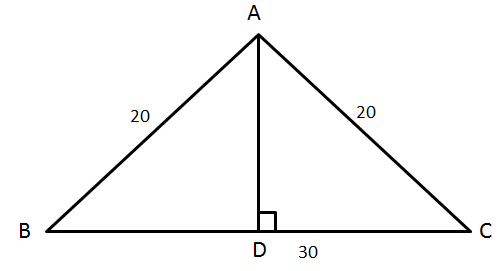{RHS congruence; AD is common, AB = AC}
DC = 15
AD2 + DC 2 = AC2
AD2 + 15 2 = 202
Area of the triangle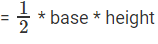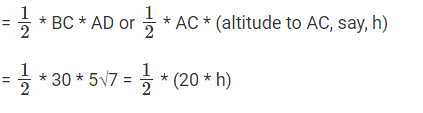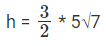h = 7.5√7

The question is " Find the altitude to side AC of triangle with side AB = 20 cm, AC = 20 cm, BC = 30 cm."

##### Hence, the answer is 7.5√7.

Choice C is the correct answer.

Question 13: ABCDEF is a regular hexagon inscribed inside a circle. If the shortest diagonal of the hexagon is of length 3 units, what is the area of the shaded region.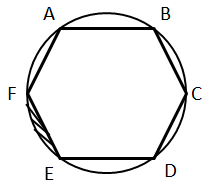A. 1/6(3π − (9√3)/2)

B. 1/6(2π − (6√3)/2)

C. 1/6(3π − (8√3)/2)

D. 1/6(6π − (15√3)/2)

Explanation.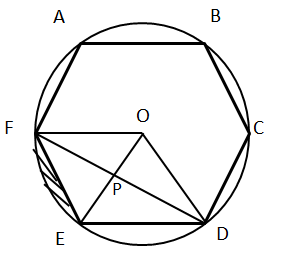Let side of regular hexagon be a.
The shortest diagonal will be of length a√3. Why?
A regular hexagon is just 6 equilateral triangles around a point. The shortest diagonal is FD.
FD = FP + PD
△FOE is equilateral and so is △ EOD.
Diagonal FD can be broken as FP + PD, both of which are altitude of equilateral s.
FP = (√3a)/2
FD = √3a = shortest diagonal
The question tells us that the shortest diagonal measures 3 cm.
√3a = 3 ⇒ a = √3
Area of hexagon = (√3a2)/4 * 6
Area of circle – area of hexagon = π (√3)2 − √3/4 * (√3)2 * 6
= 3π − (9√3)/2
Area of shaded region = 1/(6) (area(circle) – area(hexagon))
= 1/(6)(3π − (9√3)/2)

The question is "what is the area of the shaded region?"

##### Hence, the answer is 1/(6)(3π − (9√3)/2)

Choice A is the correct answer.

Question 14: A circle of radius 5 cm has chord RS at a distance of 3 units from it. Chord PQ intersects with chord RS at T such that TS = 1/3 of RT. Find minimum value of PQ.

A. 6√3

B. 4√3

C. 8√3

D. 2√3

Explanation.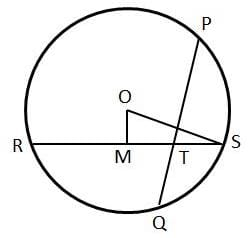OM = 3, OS = 5
MS = 4 = RM {Using Pythagoras theorem}
⇒ RS = 8 cms
TS = 1/3 of RT
TS = 1/4 of RS
TS = 2 cms
RT * TS = PT * TQ
{Intersecting Chords theorem: When there are two intersecting chords, the product of the rectangle formed by the segments of one chord is equal to the product of the rectangle formed by the segments of the other.}
6 × 2 = PT * TQ
PT * TQ = 12
By AM – GM inequality, (PT+TQ)/2 ≥ √(PT * TQ)
(PT+TQ)/2 ≥ √12
PT + TQ ≥ 2√12
⇒ PQ ≥ 2√12
Or PQ ≥ 4√3
Minimum PQ = 4√3

The question is " Find minimum value of PQ."

##### Hence, the answer is 4√3.

Choice B is the correct answer.

Question 15: Triangle has perimeter of 6 + 2√3 . One of the angles in the triangle is equal to the exterior angle of a regular hexagon another angle is equal to the exterior angle of a regular 12-sided polygon. Find area of the triangle.

A. 2√3

B. √3

C. √3/2

D. 3

Explanation.

Given, Perimeter = 6 + 2√3
One of the angles in the triangle is equal to the exterior angle of a regular hexagon which is equal to 60°
Another angle is equal to the exterior angle of a regular 12-sided polygon = 30°.
From this we can deduce that the other angle is equal to 90°.
The property of a 60-30-90 triangle is that, the sides are in the ratio √3x, x and 2x.
Therefore, Perimeter is sum of all sides = x(3+ √3)= 6 + 2√3 .
⇒ x = (6 + 2√3)/(3+ √3) = 2.
Therefore, the sides are 2√3, 2 and 4.
Area of a Right Triangle = 1/2 * Product of Perpendicular sides = 1/2 * 2 * 2√3 = 2√3 .

The question is "Perimeter of a △ with integer sides is equal to 15. How many such triangles are possible?"

##### Hence, the answer is 2√3.

Choice A is the correct answer.

Question 16: Area of a Rhombus of perimeter 56 cms is 100 sq cms. Find the sum of the lengths of its diagonals.

A. 33.40

B. 34.40

C. 31.20

D. 32.30

Explanation.

Given, Perimeter = 56 and area = 100.
Let the side of the rhombus be “a”, then 4a = 56 => a = 14.
Area of Rhombus = Half the product of its diagonals. Let the diagonals be d1 and d2 respectively.
1/2 * d1 * d2 = 100 => d1 * d2 = 200. By Pythagoras theorem, (d1)2 + (d2)2 = 4a2
⇒ (d1)2 + (d2)2 = 4*196 = 784.
(d1)2 + (d2)2 + 2d1 * d2 = (d1+ d2)2 = 784 +2*200 = 1184 => (d1+ d2) = √1184 = 34.40
Therefore, sum of the diagonals is equal to 34.40 cm .

The question is " Find the sum of the lengths of its diagonals."

##### Hence, the answer is 34.40.

Choice B is the correct answer.

Question 17Rhombus has a perimeter of 12 and one angle = 120°. Find its area.

A. 9 * (√3)/2

B. 3 * (√3)/2

C. 9 * √3

D. 18 * √3

Explanation.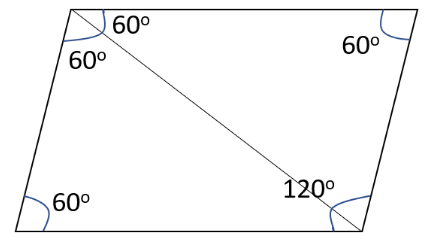Perimeter = 12.
Let the side of the rhombus be “a”, then 4a = 12 => a = 3.
One angle = 120°.
Adjacent angles of a rhombus are supplementary. Therefore, the other angle = 60°.
Diagonals of a rhombus bisect each other, therefore, ∠DAC = 60° ∠DCA =60° ∠BAC =60°
Therefore, Triangle DAC and BAC are equilateral triangles.
Therefore, Area of Rhombus = 2 * Area of the Equilateral Triangle
= 2 * (√3/4) * a2 = (√3/2) * 9 = 9 * (√3/2).

The question is " Find the area of the rhombus"

##### Hence, the answer is 9 * (√3/2).

Choice A is the correct answer.

Question 18: Circle with center O and radius 25 cms has a chord AB of length of 14 cms in it. Find the area of triangle AOB

A. 144 cm2

B. 121 cm2

C. 156 cm2

D. 168 cm2

Explanation.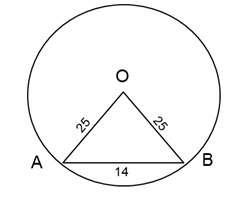Given :
Radius of the circle = 25 cm
Center is O
Length of Chord AB = 14 cm
Therefore, △ AOB will be an isosceles triangle with 2 sides OA and OB as 25 cm and side AB as 14 cm.
For an isosceles triangle with the equal sides as 'a' and base as 'b' then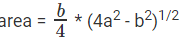Hence Area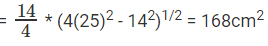The question is "Find the area of triangle AOB."

##### Hence, the answer is 168 cm2.

Choice D is the correct answer.

Question 19: Two mutually perpendicular chords AB and CD intersect at P. AP = 4, PB = 6, CP = 3. Find radius of the circle.

A. 31.25(1/2)

B. 37.5(1/2)

C. 26(1/2)

D. 52(1/2)

Explanation.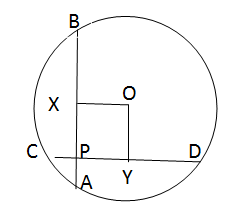When 2 chords AB and CD intersect at P then AP * PB = CP * PD
Hence 4 * 6 = 3 * PD
Thus, PD = 8
Now AB = AP + PB = 10
And CD = CP + PD
Thus, CD = 11
Consider the circle with center O.
Drop a perpendicular from O to chord AB and CD.
This will bisect the chords at X and Y i.e AX=XB and CY = YD.
Here AX = AP + PX i.e 5 = 4 + PX
PX = 1
Similarly, since CD = 11, PY+CP+YD = 11,
⇒ PY = 11-3-5.5 = 2.5.
PY = OX and PX = OY.
So, PXOY will from a rectangle as seen in the figure.
Now consider the triangle BOX, it is a right triangle where OB is the radius.
XB = 5, OX = 2.5
Then OB = ( OX2 + XB2 )1/2 OB = 31.251/2 Thus radius = 31.251/2 The radius can also be found out using the triangle YOD.

The question is "Find radius of the circle."

##### Hence, the answer is 31.251/2.

Choice A is the correct answer.

Question 20: Triangle ABC has angles A = 60° and B = 70°. The incenter of this triangle is at I. Find angle BIC.

A. 90°

B. 130°

C. 80°

D. 120°

Explanation.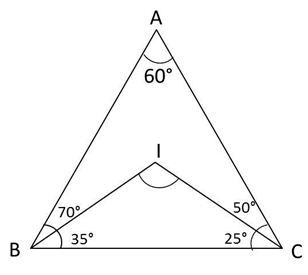A + B + C = 180 in a triangle
Hence C = 180 – 130 = 50°
Incenter is the meeting point of angle bisectors. BIC will form a triangle.
In this triangle B = 35, C = 25
Hence BIC or I = 180 – (35 + 25) = 120°

The question is "Find angle BIC."

##### Hence, the answer is 120°.

Choice D is the correct answer.

The document Geometry Questions with Answer- 2 | CSAT Preparation - UPSC is a part of the UPSC Course CSAT Preparation.
All you need of UPSC at this link: UPSC

## CSAT Preparation

197 videos|151 docs|200 tests

## FAQs on Geometry Questions with Answer- 2 - CSAT Preparation - UPSC

 1. What are the different types of angles in geometry?Ans. In geometry, there are several types of angles. These include acute angles, which measure less than 90 degrees; obtuse angles, which measure more than 90 degrees but less than 180 degrees; right angles, which measure exactly 90 degrees; and straight angles, which measure exactly 180 degrees.
 2. How can I determine the area of a triangle?Ans. To find the area of a triangle, you can use the formula A = (base x height) / 2. Simply multiply the length of the base of the triangle by the height and divide the result by 2. The resulting value will give you the area of the triangle.
 3. What is the Pythagorean theorem?Ans. The Pythagorean theorem is a fundamental concept in geometry that relates to right triangles. It states that in a right triangle, the square of the length of the hypotenuse (the side opposite the right angle) is equal to the sum of the squares of the lengths of the other two sides. This theorem is expressed as a^2 + b^2 = c^2, where a and b are the lengths of the legs of the triangle, and c is the length of the hypotenuse.
 4. How can I calculate the circumference of a circle?Ans. The circumference of a circle can be calculated using the formula C = 2πr, where C represents the circumference and r represents the radius of the circle. Alternatively, you can use the formula C = πd, where d represents the diameter of the circle. Simply substitute the values for the radius or diameter into the formula to find the circumference.
 5. What is the difference between a polygon and a polyhedron?Ans. In geometry, a polygon is a two-dimensional shape with straight sides that are connected to form a closed figure. Examples of polygons include triangles, quadrilaterals, pentagons, and hexagons. On the other hand, a polyhedron is a three-dimensional shape with flat faces, straight edges, and vertices. Examples of polyhedra include cubes, pyramids, and prisms. The main difference between the two is the number of dimensions they occupy.

## CSAT Preparation

197 videos|151 docs|200 testsExplore Courses for UPSC exam### How to Prepare for UPSC

Read our guide to prepare for UPSC which is created by Toppers & the best Teachers
Signup to see your scores go up within 7 days! Learn & Practice with 1000+ FREE Notes, Videos & Tests.
10M+ students study on EduRev
Track your progress, build streaks, highlight & save important lessons and more!
Related Searches

,

,

,

,

,

,

,

,

,

,

,

,

,

,

,

,

,

,

,

,

,

;